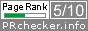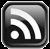LEARN MS-ACCESS TIPS AND TRICKS

Learn Microsoft Access Advanced Programming Techniques, Tips and Tricks.

Introduction

Are you afraid of learning Computer's own language, the Binary Numbering System.  I suppose you are not!  If you are a Computer Programmer or intend to become one then I suggest you better learn it.  No, you are not going to write programs in Binary Numbers, but sooner or later you will come across references to binary, Octal and Hexadecimal Numbers.  If you don't like surprises then better try getting some general understanding of this Numbering System.

I think, that is enough to keep you interested in.  It is not that hard to learn.  In fact, if you look at few simple rules that goes with the Decimal Numbering System then you can devise any numbering system that you like, if others understand it and accept it.

Few Simple Rules that Govern the Decimal Numbering System.

Let us look at few simple rules that goes with the Decimal Numbering System, that we are already familiar with.

The Decimal Numbering System.

1. Decimal Numbering System's Base value is 10 or is known as Base-10 Numbering System.
2. Base-10 Numbering System has 10 digits to express quantities; 0 to 9 and the highest digit value is 9, i.e. one less than the Base value 10.
3. Any value more than 9 is expressed in multiples of 10.

Note: Keep this simple rule in mind: Any new numbering System that you create using a particular value as Base, the number of digits in that system will be equal to the base value and the highest value of the digit in that system will be one less than the base value. We will see this when we learn some other numbering systems used in Computers (Octal & Hexadecimal Systems).

4. Decimal value 10 cannot be written with a single digit, instead we put a zero at the unit's position and write 1 in the 10th position. So, decimal Value 10 = 101 x 1 + 0 or 10 x 1 + 0.

Let us create a table to see how each digit value is calculated and added up to the decimal quantity.

 106 105 104 103 102 101 100 1,000,000 100,000 10,000 1,000 100 10 1 2 5 5

2 x 102   OR   2 x 10 x 10   OR  2 x 100  = 200

5 x 101    OR  5 x 10                              =  50

5 x 100    OR  5 x 1                                 =  5

=======

255

=======

Going by the above rules we can devise any numbering system.  For example, if we devise a Numbering System with Base-8 then this will have digits 0 to 7; the highest single digit value is one less than the base value 8 and this numbering system don't have digit 8 or 9.  In fact this Numbering System is already there in the computer world and we will learn it after going through the Binary Numbering System.

Binary Numbering System.

By keeping the above simple rules in mind we will learn Binary or Base-2 Numbering System.  This Numbering System have only two digits 0 and 1 (highest digit value is 1, i.e. one less than the base value 2) to write any Decimal value in Binary form.

First, let us create a Binary Table similar to the decimal table shown above so that converting Decimal Number to Binary and vice versa is easy.

 215 214 213 212 211 210 29 28 27 26 25 24 23 22 21 20 32,768 16,384 8,192 4,096 2,048 1,024 512 256 128 64 32 16 8 4 2 1

In the above table each digit position value is given in the second row. For example, if you put 1 below the value 1024 and fill the other slots to the right with all zeroes then the value of Binary Number 10000000000 is 1024 (or 1K or 210). If you put 1 replacing the rightmost 0 (10000000001) then the Binary Value becomes 1024+1 = 1025.

Let us try converting the small decimal number 10 to binary.

Method-1:

1. Find the highest integer value in the table above that can be subtracted from decimal number 10? 8 is the highest value that can be taken from the above table.
2. Subtract 8 from 10 and find the result.

10

-8

====

2

====

3. Put 1 under the slot of value 8 in the binary table.
4.

 215 214 213 212 211 210 29 28 27 26 25 24 23 22 21 20 32,768 16,384 8,192 4,096 2,048 1,024 512 256 128 64 32 16 8 4 2 1 1

5. Now, we have 2 as balance and the next binary position value is 4.  4 cannot be subtracted from 2, so put a 0 under the value 4.
6.

 215 214 213 212 211 210 29 28 27 26 25 24 23 22 21 20 32,768 16,384 8,192 4,096 2,048 1,024 512 256 128 64 32 16 8 4 2 1 1 0

7. Next value 2 can be subtracted from the balance value 2 above.

2

-2

=====

0

=====

8. Put 1 under the value 2 in the binary table. There is nothing left to calculate binary value so put a 0 in the unit position of binary table. So the result of Decimal Number 10 in binary form is as given below:

 215 214 213 212 211 210 29 28 27 26 25 24 23 22 21 20 32,768 16,384 8,192 4,096 2,048 1,024 512 256 128 64 32 16 8 4 2 1 1 0 1 0

Cross-Checking the Result.

You can quickly cross check whether the binary number is correct to the decimal number by adding up the values in the second row wherever one is there in the third row;  8+2  is 10.

It is not always convenient to build the above table every time when we want to convert a decimal number to binary.  Instead we can do it with a simple calculation.  Let us convert decimal number 10 to binary with this new method.

Method-2:

1. Divide the decimal number by 2 and get the remainder value (always integer division and the remainder is 1 or 0) and start building the binary value from right to left with the remainder value.
2.

10/2 = Quotient = 5, Remainder=0

 215 214 213 212 211 210 29 28 27 26 25 24 23 22 21 20 32,768 16,384 8,192 4,096 2,048 1,024 512 256 128 64 32 16 8 4 2 1 0

3. Each time take the Quotient Value from the previous division and divide it by 2 again.
4.

5/2 = Quotient = 2, Remainder = 1

 215 214 213 212 211 210 29 28 27 26 25 24 23 22 21 20 32,768 16,384 8,192 4,096 2,048 1,024 512 256 128 64 32 16 8 4 2 1 1 0

2/2 = Quotient=1, Remainder = 0

 215 214 213 212 211 210 29 28 27 26 25 24 23 22 21 20 32,768 16,384 8,192 4,096 2,048 1,024 512 256 128 64 32 16 8 4 2 1 0 1 0

1/2 = Quotient = 0, Remainder=1

 215 214 213 212 211 210 29 28 27 26 25 24 23 22 21 20 32,768 16,384 8,192 4,096 2,048 1,024 512 256 128 64 32 16 8 4 2 1 1 0 1 0

If you understood this simple numbering system so far then try converting some numbers bigger than what we have tried. As you can see from the binary table above the highest value we have plotted on the table above is 32768 (215) but you can try converting any value below the decimal Number 65536 with the above table.

If you need a sample number then try converting decimal number 255 into binary.

Share:

1.[...] ToolbarViral PDF eBooks - turn your PDF documents into a viral sales force  Related Links:Learn Binary Numbering SystemConverting From Decimal to Binary, Octal, and HexadecimalDigital Electronics MCQ Free Download PDF [...]

2.Hi, and thanks for the comment on my post on understanding binary! I think yours is intended for the more confident reader, whereas mine has the goal of giving a brief introduction to those who feel they are in some way not as mathematical. Yours may well be a good place to take a step up from mine (similarly, mine may be a good place to start for any who find yours starts off too high!)

Just in case, mine's here: http://mathsqs.blogspot.com/2010/10/how-does-binary-work.html

3.Thanks for the review TeaKayB.

Regards,

4.Here is a good site for people wanting to learn binary and hexadecimal.
http://justwebware.com/bitwise/bitwise.html

Comments are subject to moderation before publishing.

TranslateSubscribe in a readerYour email address:

Delivered by FeedBurner

Featured Post

ROUNDDOWN Function of Excel

We have introduced ROUNDUP() Function of Excel in Microsoft Access earlier.  It works like ROUND() Function except that the number is alway...